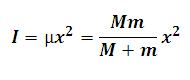# Moment of Inertia of a Two Point Reduced Mass Sysytem

This Calctown Calculator calculates the Moment of Inertia of a Two Point Reduced Mass System.

kg
kg
m

#### Result

kg-m2where

I = Moment of Inertia

μ = Reduced mass of given two point system M and m

x = distance between two given point masses